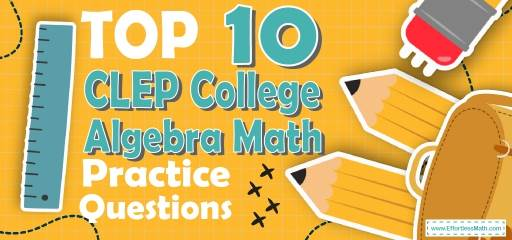# Top 10 CLEP College Algebra Practice QuestionsPreparing for the CLEP College Algebra test? The best way to prepare for your CLEP Algebra test is to work through as many CLEP Algebra practice questions as possible. Here are the top 10 CLEP College Algebra practice questions to help you review the most important CLEP College Algebra concepts. These CLEP Algebra practice questions are designed to cover mathematics concepts and topics that are found on the actual test. The questions have been fully updated to reflect the latest 2021 CLEP College Algebra guidelines. Answers and full explanations are provided at the end of the post.

Start your CLEP College Algebra test prep journey right now with these sample CLEP College Algebra questions.

## CLEP College Algebra Practice Questions

1- Which of the following points lies on the line $$2x+4y=6$$?

A. $$(2,1)$$

B. $$(-1,2)$$

C. $$(-2,2)$$

D. $$(2,2)$$

E. $$(2,8)$$

2- Point A lies on the line with equation $$y-3=2(x+5)$$. If the $$x$$-coordinate of A is $$8$$, what is the $$y$$-coordinate of A?

A. 14

B. 16

C. 22

D. 29

E. 31

3- Right triangle ABC has two legs of lengths 5 cm (AB) and 12 cm (AC). What is the length of the third side (BC)?

A. 4 cm

B. 6 cm

C. 8 cm

D. 13 cm

E. 20 cm

4- The ratio of boys to girls in a school is 2:3. If there are 500 students in a school, how many boys are in the school?

A. 540

B. 360

C. 300

D. 280

E. 200

5- $$(7x+2y)(5x+2y) =$$?

A. $$2x^2+14xy+2y^2$$

B. $$2x^2+4xy+2y^2$$

C. $$7x^2+14xy+y^2$$

D. $$10x^2+14xy+4y$$

E. $$35x^2+24xy+4y^2$$

6- Which of the following expressions is equivalent to $$5x (4+2y)$$?

A. $$x+10xy$$

B. $$5x+5xy$$

C. $$20xy+2xy$$

D. $$20x+5xy$$

E. $$20x+10xy$$

7- If $$y=5ab+3b^3$$, what is y when $$a=2$$ and $$b=3$$?

A. 24

B. 31

C. 36

D. 51

E. 111

8- From the figure, which of the following must be true? (figure not drawn to scale)

A. $$y = z$$

B. $$y = 5x$$

C. $$y≥x$$

D. $$y+4x=z$$

E. $$y>x$$

9- What is the solution to the following inequality?
$$|x-10|≤3$$

A. $$x≥13 ∪ x≤7$$

B. $$7≤x≤13$$

C. $$x≥13$$

D. $$x≤7$$

E. Set of real numbers

10- Two-third of 15 is equal to $$\frac{2}{5}$$ of what number?

A. 12

B. 20

C. 25

D. 60

E. 90

## Best CLEP College Algebra Prep Resource for 2022

1- B
Plug in each pair of number in the equation:
$$(2, 1): 2 (2) + 4 (1) = 8$$ Nope!
$$(–1, 2): 2 (–1) + 4 (2) = 6$$ Bingo!
$$(–2, 2): 2 (–2) + 4 (2) = 4$$ Nope!
$$(2, 2): 2 (2) + 4 (2) = 12$$ Nope!
$$(2, 8): 2 (2) + 4 (8) = 36$$ Nope!

2- D
Here we can substitute 8 for $$x$$ in the equation. Thus, $$y-3=2(8+5), y-3=26$$
Adding 3 to both side f the equation: $$y=26+3, y=29$$

3- D
Use Pythagorean Theorem: $$a^2+b^2=c^2⇒5^2+12 ^2=c^2⇒169=c^2⇒c=13$$

4- E
The ratio of boys to girls is 2:3. Therefore, there are 2 boys out of 5 students. To find the answer, first, divide the total number of students by 5, then multiply the result by 2.
$$500÷5=100 ⇒100 × 2=200$$

5- E
Use FOIL (First, Out, In, Last).$$(7x+2y)(5x+2y)=$$
$$35x^2+14xy+10xy+4y^2=$$
$$35x^2+ 24xy+4y^2$$

6- E
Use distributive property: $$5x(4+2y)=20x+10xy$$

7- E
$$y=5ab+3b^3$$. Plug in the values of a and b in the equation: $$a=2$$ and $$b=3$$.
$$y=5 (2)(3)+3 (3)^3=30+3(27)=30+81=111$$

8- D
$$x$$ and $$z$$ are colinear. $$y$$ and $$5x$$ are colinear. Therefore,
$$x+z=y+5x$$,subtract $$x$$ from both sides,then,$$z=y+4x$$

9- B
$$|x-10|≤3→-3≤x-10≤3→-3+10≤x-10+10≤3+10→7≤x≤13$$

10- C
Let $$x$$ be the number. Write the equation and solve for $$x$$. $$\frac{2}{3} ×15= \frac{2}{5}$$. $$x⇒\frac{2×15}{3}=\frac{2x}{5 }$$, use cross multiplication to solve for $$x$$. $$5×30=2x×3⇒150=6x⇒x=25$$

Looking for the best resource to help you succeed on the CLEP College Algebra test?

## The Best Books to Ace the CLEP College Algebra Test

### What people say about "Top 10 CLEP College Algebra Practice Questions - Effortless Math: We Help Students Learn to LOVE Mathematics"?

No one replied yet.

X
23% OFF

Limited time only!

Save Over 23%

SAVE $5 It was$21.99 now it is \$16.99